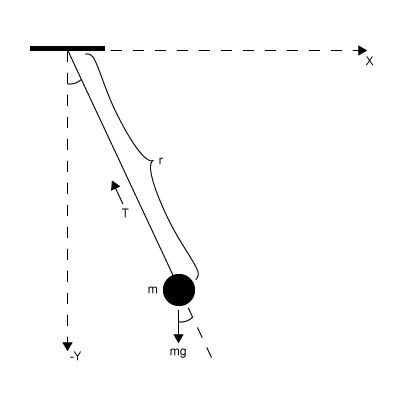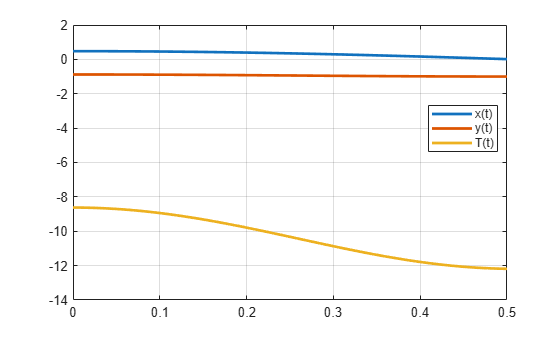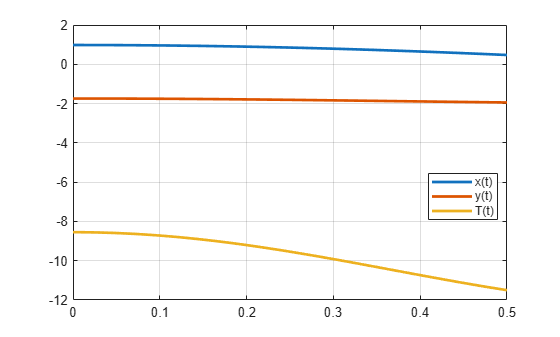# Solve Differential Algebraic Equations (DAEs)

This example show how to solve differential algebraic equations (DAEs) by using MATLAB® and Symbolic Math Toolbox™.

Differential algebraic equations involving functions, or state variables, $\mathit{x}\left(\mathit{t}\right)=\left[{\mathit{x}}_{1}\left(\mathit{t}\right),...,{\mathit{x}}_{\mathit{n}}\left(\mathit{t}\right)\right]$ have the form

`$F\left(t,x\left(t\right),\underset{}{\overset{˙}{x}}\left(t\right)\right)=0$`

where $\mathit{t}$ is the independent variable. The number of equations $\mathit{F}=\left[{\mathit{F}}_{1},...,{\mathit{F}}_{\mathit{n}}\right]$ must match the number of state variables $\mathit{x}\left(\mathit{t}\right)=\left[{\mathit{x}}_{1}\left(\mathit{t}\right),...,{\mathit{x}}_{\mathit{n}}\left(\mathit{t}\right)\right]$.

Because most DAE systems are not suitable for direct input to MATLAB solvers, such as `ode15i`, first convert them to a suitable form by using Symbolic Math Toolbox functionality. This functionality reduces the differential index (number of differentiations needed to reduce the system to ODEs) of the DAEs to 1 or 0, and then converts the DAE system to numeric function handles suitable for MATLAB solvers. Then, use MATLAB solvers, such as `ode15i`, `ode15s`, or `ode23t`, to solve the DAEs.

Solve your DAE system by completing these steps.

### Step 1: Specify Equations and Variables

The following figure shows the DAE workflow by solving the DAEs for a pendulum.The state variables are:

• Horizontal position of pendulum $\mathit{x}\left(\mathit{t}\right)$

• Vertical position of pendulum $\mathit{y}\left(\mathit{t}\right)$

• Force preventing pendulum from flying away $\mathit{T}\left(\mathit{t}\right)$

The variables are:

• Pendulum mass $\mathit{m}$

• Pendulum length $\mathit{r}$

• Gravitational constant $\mathit{g}$

The DAE system of equations is:

`$\begin{array}{l}m\frac{{d}^{2}x}{d{t}^{2}}=T\left(t\right)\frac{x\left(t\right)}{r}\\ m\frac{{d}^{2}y}{d{t}^{2}}=T\left(t\right)\frac{y\left(t\right)}{r}-mg\\ x{\left(t\right)}^{2}+y{\left(t\right)}^{2}={r}^{2}\end{array}$`

Specify independent variables and state variables by using `syms`.

`syms x(t) y(t) T(t) m r g`

Specify equations by using the == operator.

```eqn1 = m*diff(x(t), 2) == T(t)/r*x(t); eqn2 = m*diff(y(t), 2) == T(t)/r*y(t) - m*g; eqn3 = x(t)^2 + y(t)^2 == r^2; eqns = [eqn1 eqn2 eqn3];```

Place the state variables in a column vector. Store the number of original variables for reference.

```vars = [x(t); y(t); T(t)]; origVars = length(vars);```

### Step 2: Reduce Differential Order

2.1 (Optional) Check Incidence of Variables

This step is optional. You can check where variables occur in the DAE system by viewing the incidence matrix. This step finds any variables that do not occur in your input and can be removed from the `vars` vector.

Display the incidence matrix by using `incidenceMatrix`. The output of `incidenceMatrix` has a row for each equation and a column for each variable. Because the system has three equations and three state variables, `incidenceMatrix` returns a `3`-by-`3` matrix. The matrix has `1`s and `0`s, where `1`s represent the occurrence of a state variable. For example, the `1` in position `(2,3)` means the second equation contains the third state variable `T(t)`.

`M = incidenceMatrix(eqns,vars)`
```M = 3×3 1 0 1 0 1 1 1 1 0 ```

If a column of the incidence matrix is all `0`s, then that state variable does not occur in the DAE system and should be removed.

2.2 Reduce Differential Order

The differential order of a DAE system is the highest differential order of its equations. To solve DAEs using MATLAB, the differential order must be reduced to `1`. Here, the first and second equations have second-order derivatives of `x(t)` and `y(t)`. Thus, the differential order is `2`.

Reduce the system to a first-order system by using `reduceDifferentialOrder`. The `reduceDifferentialOrder` function substitutes derivatives with new variables, such as `Dxt(t)` and `Dyt(t)`. The right side of the expressions in `eqns` is `0`.

`[eqns,vars] = reduceDifferentialOrder(eqns,vars)`
```eqns =  ```
```vars =  $\left(\begin{array}{c}x\left(t\right)\\ y\left(t\right)\\ T\left(t\right)\\ \mathrm{Dxt}\left(t\right)\\ \mathrm{Dyt}\left(t\right)\end{array}\right)$```

### Step 3: Check and Reduce Differential Index

3.1 Check Differential Index of System

Check the differential index of the DAE system by using `isLowIndexDAE`. If the index is `0` or `1`, then `isLowIndexDAE` returns logical `1` (`true`) and you can skip step 3.2 and go to Step 4. Convert DAE Systems to MATLAB Function Handles. Here, `isLowIndexDAE` returns logical `0 `(`false`), which means the differential index is greater than `1` and must be reduced.

`isLowIndexDAE(eqns,vars)`
```ans = logical 0 ```

3.2 Reduce Differential Index with `reduceDAEIndex`

To reduce the differential index, the `reduceDAEIndex` function adds new equations that are derived from the input equations, and then replaces higher-order derivatives with new variables. If `reduceDAEIndex` fails and issues a warning, then use the alternative function `reduceDAEToODE` as described in the workflow Solve Semilinear DAE System.

Reduce the differential index of the DAEs described by `eqns` and `vars`.

`[DAEs,DAEvars] = reduceDAEIndex(eqns,vars)`
```DAEs =  ```
```DAEvars =  $\left(\begin{array}{c}x\left(t\right)\\ y\left(t\right)\\ T\left(t\right)\\ \mathrm{Dxt}\left(t\right)\\ \mathrm{Dyt}\left(t\right)\\ \mathrm{Dytt}\left(t\right)\\ \mathrm{Dxtt}\left(t\right)\\ {\mathrm{Dxt}}_{1}\left(t\right)\\ {\mathrm{Dyt}}_{1}\left(t\right)\\ \mathrm{Dxt1t}\left(t\right)\end{array}\right)$```

If `reduceDAEIndex` issues an error or a warning, use the alternative workflow described in Solve Semilinear DAE System.

Often, `reduceDAEIndex` introduces redundant equations and variables that can be eliminated. Eliminate redundant equations and variables using `reduceRedundancies`.

`[DAEs,DAEvars] = reduceRedundancies(DAEs,DAEvars)`
```DAEs =  ```
```DAEvars =  $\left(\begin{array}{c}x\left(t\right)\\ y\left(t\right)\\ T\left(t\right)\\ \mathrm{Dxt}\left(t\right)\\ \mathrm{Dyt}\left(t\right)\\ \mathrm{Dytt}\left(t\right)\\ \mathrm{Dxtt}\left(t\right)\end{array}\right)$```

Check the differential index of the new system. Now, `isLowIndexDAE` returns logical `1` (`true`), which means that the differential index of the system is `0` or `1`.

`isLowIndexDAE(DAEs,DAEvars)`
```ans = logical 1 ```

### Step 4: Convert DAE Systems to MATLAB Function Handles

This step creates function handles for the MATLAB ODE solver `ode15i`, which is a general purpose solver. To use specialized mass matrix solvers such as `ode15s` and `ode23t`, see Solve DAEs Using Mass Matrix Solvers and Choose an ODE Solver.

`reduceDAEIndex` outputs a vector of equations in `DAEs` and a vector of variables in `DAEvars`. To use `ode15i`, you need a function handle that describes the DAE system.

First, the equations in `DAEs` can contain symbolic parameters that are not specified in the vector of variables `DAEvars`. Find these parameters by using `setdiff` on the output of `symvar` from `DAEs` and `DAEvars`.

```pDAEs = symvar(DAEs); pDAEvars = symvar(DAEvars); extraParams = setdiff(pDAEs,pDAEvars)```
`extraParams = $\left(\begin{array}{ccc}g& m& r\end{array}\right)$`

The extra parameters that you need to specify are the mass `m`, radius `r`, and gravitational constant `g`.

Create the function handle by using `daeFunction`. Specify the extra symbolic parameters as additional input arguments of `daeFunction`.

`f = daeFunction(DAEs,DAEvars,g,m,r);`

The rest of the workflow is purely numerical. Set the parameter values and create the function handle for `ode15i`.

```g = 9.81; m = 1; r = 1; F = @(t,Y,YP) f(t,Y,YP,g,m,r);```

### Step 5: Find Initial Conditions for Solvers

The `ode15i` solver requires initial values for all variables in the function handle. Find initial values that satisfy the equations by using the MATLAB `decic` function. `decic` accepts guesses (which might not satisfy the equations) for the initial conditions and tries to find satisfactory initial conditions using those guesses. `decic` can fail, in which case you must manually supply consistent initial values for your problem.

First, check the variables in `DAEvars`.

`DAEvars`
```DAEvars =  $\left(\begin{array}{c}x\left(t\right)\\ y\left(t\right)\\ T\left(t\right)\\ \mathrm{Dxt}\left(t\right)\\ \mathrm{Dyt}\left(t\right)\\ \mathrm{Dytt}\left(t\right)\\ \mathrm{Dxtt}\left(t\right)\end{array}\right)$```

Here, `Dxt(t)` is the first derivative of `x(t)`, `Dytt(t)` is the second derivative of `y(t)`, and so on. There are 7 variables in a `7`-by-`1` vector. Therefore, guesses for initial values of variables and their derivatives must also be `7`-by-`1` vectors.

Assume the initial angular displacement of the pendulum is 30° or `pi/6`, and the origin of the coordinates is at the suspension point of the pendulum. Given that we used a radius `r` of `1`, the initial horizontal position `x(t)` is `r*sin(pi/6)`. The initial vertical position `y(t)` is `-r*cos(pi/6)`. Specify these initial values of the variables in the vector `y0est`.

Arbitrarily set the initial values of the remaining variables and their derivatives to `0`. These are not good guesses. However, they suffice for this problem. In your problem, if `decic` errors, then provide better guesses and refer to `decic`.

```y0est = [r*sin(pi/6); -r*cos(pi/6); 0; 0; 0; 0; 0]; yp0est = zeros(7,1);```

Create an option set that specifies numerical tolerances for the numerical search.

`opt = odeset('RelTol', 10.0^(-7),'AbsTol',10.0^(-7));`

Find consistent initial values for the variables and their derivatives by using `decic`.

`[y0,yp0] = decic(F,0,y0est,[],yp0est,[],opt)`
```y0 = 7×1 0.4771 -0.8788 -8.6214 0 -0.0000 -2.2333 -4.1135 ```
```yp0 = 7×1 0 -0.0000 0 0 -2.2333 0 0 ```

### Step 6: Solve DAEs Using `ode15i`

Solve the system integrating over the time span `0``t``0.5`. Add the grid lines and the legend to the plot.

```[tSol,ySol] = ode15i(F,[0 0.5],y0,yp0,opt); plot(tSol,ySol(:,1:origVars),'LineWidth',2) for k = 1:origVars S{k} = char(DAEvars(k)); end legend(S,'Location','Best') grid on```Solve the system for different parameter values by setting the new value and regenerating the function handle and initial conditions.

Set `r` to `2` and regenerate the function handle and initial conditions.

```r = 2; F = @(t,Y,YP)f(t,Y,YP,g,m,r); y0est = [r*sin(pi/6); -r*cos(pi/6); 0; 0; 0; 0; 0]; [y0,yp0] = decic(F,0,y0est,[],yp0est,[],opt);```

Solve the system for the new parameter value.

```[tSol,y] = ode15i(F,[0 0.5],y0,yp0,opt); plot(tSol,y(:,1:origVars),'LineWidth',2) for k = 1:origVars S{k} = char(DAEvars(k)); end legend(S,'Location','Best') grid on```